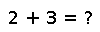issubset

``````..  issubset(A, S) -> Bool
⊆(A,S) -> Bool

Return ``true`` if ``A`` is a subset of or equal to ``S``.``````

Examples

``````julia> foo = [1:3];

julia> issubset(foo, [1:10])
true

julia> issubset(foo, [1:2])
false

julia> issubset(foo, [3:-1:1])
true``````

Checking you are not a robot: